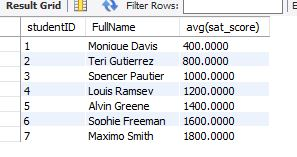# A guide to the SQL Avg Function

A guide to the SQL Avg Function
0

## SQL Average (AVG) Function

“Average” is an Aggregate (Group By) Function. It’s used to calculate the average of a numeric column from the set of rows returned by a SQL statement.

Here is the syntax for using the function:

``````select groupingField, avg(num_field)
from table1
group by groupingField
``````

Here’s an example using the student table:

``````select studentID, FullName, avg(sat_score)
from student
group by studentID, FullName;
``````